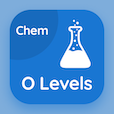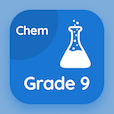Cambridge O Level Courses

O Level Chemistry Prep Tests

O Level Chemistry Tests

The e-Book Valency and Chemical Formula Multiple Choice Questions (MCQ Quiz), Valency and Chemical Formula quiz answers PDF to learn online courses, o level chemistry tests. Study Chemical Formulae and Equations Multiple Choice Questions and Answers (MCQs), Valency and Chemical Formula quiz questions for schools that offer online bachelor degrees. The e-Book "Valency and Chemical Formula MCQ" App Download: valency and chemical formula, chemical symbols test prep for GRE prep classes.

The MCQ "The chemical formula of Zinc Hydroxide is" PDF, Valency and Chemical Formula App Download (Free) with zn(oh)2, zno2, zn2(oh)3, and znoh choices for schools that offer online bachelor degrees. Practice valency and chemical formula quiz questions, download Amazon eBook (Free Sample) for ACT subject test tutoring.

## Chemistry: Valency and Chemical Formula MCQs

MCQ: The chemical formula of Zinc Hydroxide is

A) Zn(OH)2
B) ZNO2
C) Zn2(OH)3
D) ZnOH

MCQ: The empirical formula is the

A) simplest formula
B) molecular formula
C) chemical formula
D) ionic exponential formula

MCQ: All of these radicals have a Valency of 2, except

A) SO4
B) CO3
C) NH4
D) MgCO3

MCQ: In CuO (Copper Oxide), the valency of Copper is

A) +1
B) +2
C) −1
D) −2

MCQ: Formula of Ammonium Sulfate ((NH4)2SO4) is

A) NH4(SO4)2
B) NH4(HSO4)2
C) (NH4)2SO4
D) (NH3)2SO3

### Practice Tests: O Level Chemistry Exam Prep

Download O Level Chemistry Quiz App, 9th Grade Chemistry MCQs App and College Chemistry MCQ App for Android & iOS devices. These Apps include complete analytics of real time attempts with interactive assessments. Download Play Store & App Store Apps & Enjoy 100% functionality with subscriptions!